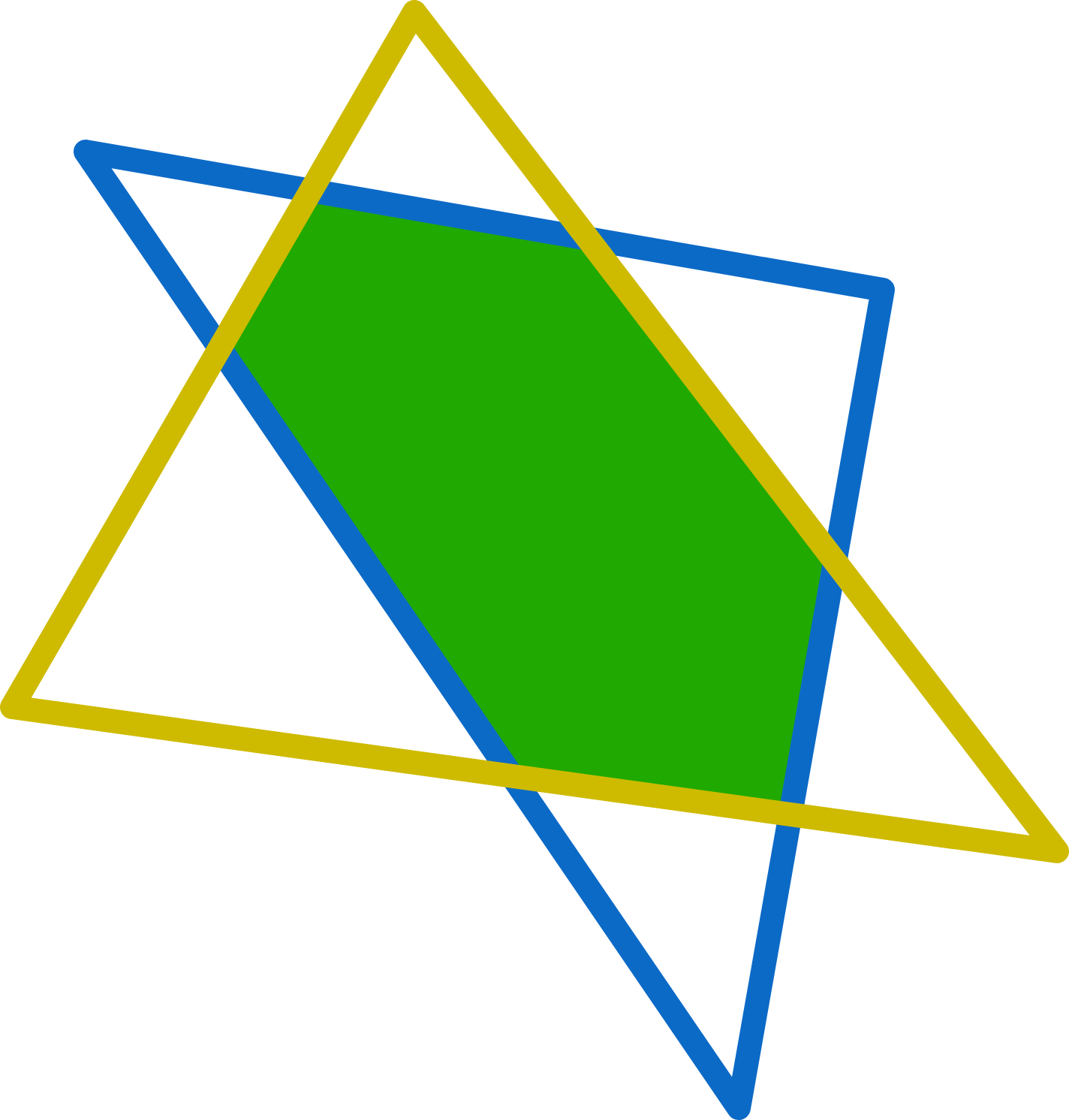# A geometry problem by Chung Kevin

Geometry Level 1If the perimeter of the yellow triangle is 20,
and the perimeter of the blue triangle is 30,
and the perimeter of the six-pointed star is 40,
what is the perimeter of the green hexagon?

×